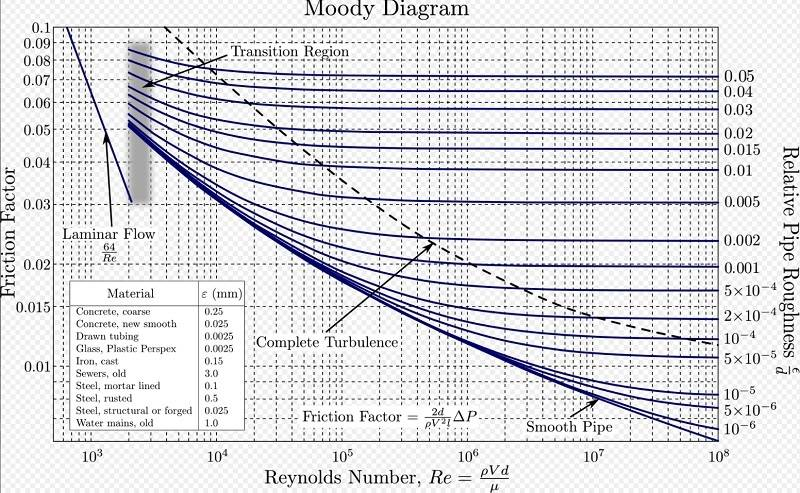# Advice on pressure drop calculation

DarkoX
TL;DR Summary
I am desperately need an advise/help on the calculation of pressure drop across the pipe.
Hello everyone,

I would appreciate any help with my task I am struggling to resolve. I need to calculate or simulate pressure drop when got the following parameters:

1. Supply pressure: 45barg.
2. Pipe Length: 80 meters
3. Pipe diameter: OD - 10mm, ID - 8mm.
4. Pipe material: stainless steel (SS316).
5. Pipe has 20 90deg bends distributed evenly across the pipe length.
6. Flow: well, it is bit more complicated as flow measured at 50 litres/min however after pressure is reduced from 45barg to 3.5barg (after pressure regulator).
7. Media: Pure N2 (nitrogen) gas.

How hard to calculate/simulate pressure drop across this pipe? Anyone can help me on this?

Much appreciated.

Mentor
The Moody Chart (search the term) has everything you need to calculate the pressure drop. The chart in Wikipedia is a good one. You will need to do some calculations. Start as follows:

1) Calculate the relative roughness. If your pipe is drawn tubing, that would be 0.0025 / 8 = 3E-4.

2) Find the nitrogen viscosity. Here is a good source: https://www.engineeringtoolbox.com/air-absolute-kinematic-viscosity-d_601.html.

3) Calculate the Reynolds number. You will need to calculate or find the density, velocity, and viscosity of the nitrogen as it is flowing through the pipe. All of the inputs to the Reynolds number must be in consistent units so that the units will cancel. A properly calculated Reynolds number is dimensionless, and you need to confirm that the units cancel in order to verify your calculation.

4) Now that you have the Reynolds number and relative roughness, find the friction factor from the Moody chart. A screen shot of the Wikipedia Moody chart is shown below.

5) Find the equivalent length of one of the pipe 90 degree bends. Add to the actual length of the pipe, and use that total (actual plus equivalent) length for all pressure drop calculations. Use search term equivalent length pipe bend.

6) Use the equation on the Moody chart to find the pressure drop. If the pressure drop is less than about 10% of the inlet absolute pressure, you are done. If the pressure drop is greater than 10% (10% of 45 barg is 4.5 barg), divide the pipe into several lengths. Calculate the pressure at the end of the first length, use that as the input to the second length, etc.

Moody Chart:You may be able find an online calculator, but it is a very good idea to verify by doing the calculation above.

•BvU and Lnewqban
Mentor
The Moody Chart (search the term) has everything you need to calculate the pressure drop. The chart in Wikipedia is a good one. You will need to do some calculations. Start as follows:

1) Calculate the relative roughness. If your pipe is drawn tubing, that would be 0.0025 / 8 = 3E-4.

2) Find the nitrogen viscosity. Here is a good source: https://www.engineeringtoolbox.com/air-absolute-kinematic-viscosity-d_601.html.

3) Calculate the Reynolds number. You will need to calculate or find the density, velocity, and viscosity of the nitrogen as it is flowing through the pipe. All of the inputs to the Reynolds number must be in consistent units so that the units will cancel. A properly calculated Reynolds number is dimensionless, and you need to confirm that the units cancel in order to verify your calculation.

4) Now that you have the Reynolds number and relative roughness, find the friction factor from the Moody chart. A screen shot of the Wikipedia Moody chart is shown below.

5) Find the equivalent length of one of the pipe 90 degree bends. Add to the actual length of the pipe, and use that total (actual plus equivalent) length for all pressure drop calculations. Use search term equivalent length pipe bend.

6) Use the equation on the Moody chart to find the pressure drop. If the pressure drop is less than about 10% of the inlet absolute pressure, you are done. If the pressure drop is greater than 10% (10% of 45 barg is 4.5 barg), divide the pipe into several lengths. Calculate the pressure at the end of the first length, use that as the input to the second length, etc.

Moody Chart:
View attachment 285474

You may be able find an online calculator, but it is a very good idea to verify by doing the calculation above.
One more thing. With N2 gas, the density will be changing. So we have $$\frac{dp}{dx}=-\frac{\rho v^2}{2d}f=-\frac{(\rho v)^2}{2\rho d}f=-(Re)^2\frac{\mu^2}{2\rho d^3}f$$with $$Re=\frac{4m}{\pi d \mu}$$where m is the mass flow rate. In addition, we have $$\rho=\frac{pM}{RT}$$This leads to $$\frac{dp^2}{dx}=-(Re)^2\left(\frac{RT}{M}\right)\frac{\mu^2}{d^3}f$$So, for a compressible ideal gas, we are working with the square of the pressure, rather than the pressure to the first power.

•BvU